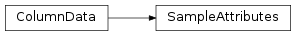# mvpa2.misc.io.base.SampleAttributes¶class `mvpa2.misc.io.base.``SampleAttributes`(source, literallabels=True, header=None)

Read and write PyMVPA sample attribute definitions from and to text files.

Attributes

 `ncolumns` Returns the number of columns. `nrows` Returns the number of rows. `nsamples` Returns the number of samples in the file.

Methods

 `clear`(() -> None.  Remove all items from D.) `copy`(() -> a shallow copy of D) `fromkeys`(...) v defaults to None. `get`((k[,d]) -> D[k] if k in D, ...) `has_key`((k) -> True if D has a key k, else False) `items`(() -> list of D’s (key, value) pairs, ...) `iteritems`(() -> an iterator over the (key, ...) `iterkeys`(() -> an iterator over the keys of D) `itervalues`(...) `keys`(() -> list of D’s keys) `pop`((k[,d]) -> v, ...) If key is not found, d is returned if given, otherwise KeyError is raised `popitem`(() -> (k, v), ...) 2-tuple; but raise KeyError if D is empty. `select_samples`(selection) Return new ColumnData with selected samples `setdefault`((k[,d]) -> D.get(k,d), ...) `tofile`(filename) Write sample attributes to a text file. `update`(([E, ...) If E present and has a .keys() method, does: for k in E: D[k] = E[k] `values`(() -> list of D’s values) `viewitems`(...) `viewkeys`(...) `viewvalues`(...)

Read PyMVPA sample attributes from disk.

Parameters: source : str Filename of an atrribute file literallabels : bool, optional Either labels are given as literal strings header : None or bool or list of str If None, [‘targets’, ‘chunks’] is assumed. Otherwise the same behavior as of `ColumnData`

Attributes

 `ncolumns` Returns the number of columns. `nrows` Returns the number of rows. `nsamples` Returns the number of samples in the file.

Methods

 `clear`(() -> None.  Remove all items from D.) `copy`(() -> a shallow copy of D) `fromkeys`(...) v defaults to None. `get`((k[,d]) -> D[k] if k in D, ...) `has_key`((k) -> True if D has a key k, else False) `items`(() -> list of D’s (key, value) pairs, ...) `iteritems`(() -> an iterator over the (key, ...) `iterkeys`(() -> an iterator over the keys of D) `itervalues`(...) `keys`(() -> list of D’s keys) `pop`((k[,d]) -> v, ...) If key is not found, d is returned if given, otherwise KeyError is raised `popitem`(() -> (k, v), ...) 2-tuple; but raise KeyError if D is empty. `select_samples`(selection) Return new ColumnData with selected samples `setdefault`((k[,d]) -> D.get(k,d), ...) `tofile`(filename) Write sample attributes to a text file. `update`(([E, ...) If E present and has a .keys() method, does: for k in E: D[k] = E[k] `values`(() -> list of D’s values) `viewitems`(...) `viewkeys`(...) `viewvalues`(...)
`nsamples`

Returns the number of samples in the file.

`tofile`(filename)

Write sample attributes to a text file.